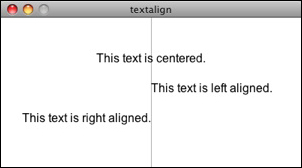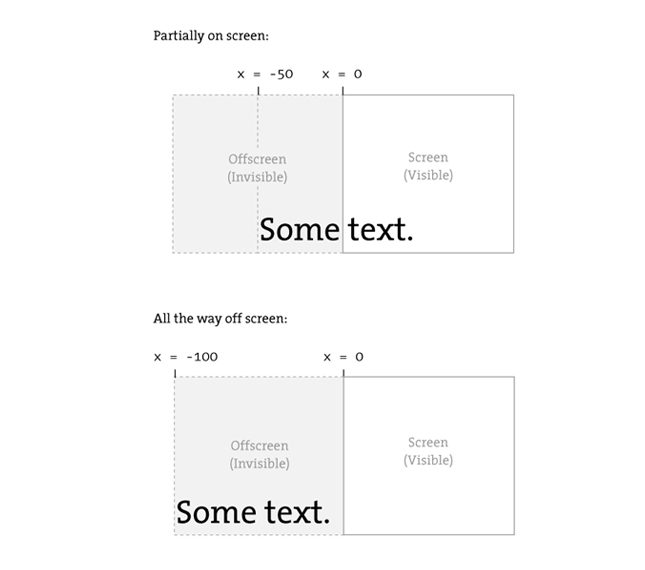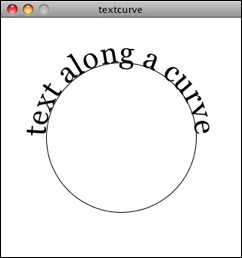# Strings and Drawing Text

### The String Class

If you are looking to display text onscreen with Processing, you've got to first become familiar with python's built-in string class. Strings are probably not a totally new concept for you, it's quite likely you've dealt with them before. For example, if you've printed some text to the message window or loaded an image from a file, you've written code like so:

```print("printing some text to the message window!")  # Printing a string
img = loadImage("filename.jpg")                     # Using a string for a file name
```

Nevertheless, although you may have used a string here and there, it's time to unleash their full potential.

### Where do we find documentation for the string class?

Although technically a Python class, because strings are so commonly used, Processing.py includes documentation in its reference. Here you'll find reference pages like the generalstring class.

This page only covers some of the available methods of the String class. The full documentation can be found on python's string page.

### What is a string?

A string, at its core, is really just a fancy way of storing a list of characters. If we didn't have the string class, we'd probably have to write some code like this:

```sometext = ['H', 'e', 'l', 'l', 'o', ' ', 'W', 'o', 'r', 'l', 'd']
```

Clearly, this would be a royal pain in the Processing behind. It's much simpler to do the following and make a string object:

```sometext = "How do I make string? Type some characters between quotation marks!"
```

It appears from the above that a string is nothing more than a list of characters in between quotes. Nevertheless, this is only the data of a string. We must remember that a string is an object with methods (which you can find on the reference page.) This is just like how we learned in the Pixels tutorial that a PImage stores both the data associated with an image as well as the functionality: copy(), loadPixels(), etc.

For example, using square brackets at the end of a string (i.e. myString) returns the individual character in the string at a given index. This is actually just a simple way of calling the strings __getitem__() method! Note that Strings are just like lists in that the first character is index #0!

```message = "some text here."
c = message
print(c)                   # Results in 'e'
```

Another useful function is len(). By calling the len() function with our string as its argument, we can retrieve the length of our string.

```message = "This String is 34 characters long."
print( len(message) )                          # Prints 34
```

We can also change a string to all uppercase using the .upper() method (.lower is also available).

```uppercase = message.upper
println(uppercase)
```

You might notice something a bit odd here. Why didn't we simply say "message.upper()" and then print "message" variable? Instead, we assigned the result of "message.upper" to a new variable with a different name -- "uppercase".

This is because a string is a special kind of object. It is immutable. An immutable object is one whose data can never be changed. Once we create a string, it stays the same for life. Anytime we want to change the string, we have to create a new one. So in the case of converting to uppercase, the method .upper() returns a copy of the string object with all caps.

Finally, let's look at the == (equality) operator. Now, strings can be compared with the "==" operator as follows:

```one = "hello"
two = "hello"
print(one == two) # True!
```

While in other languages strings have their own equality checking methods, in Python the equality check is built into the default == (equality) operator. This makes comparing strings super simple!

One other feature of string objects is concatenation, joining two strings together. Strings are joined with the "+" operator. Plus, of course, usually means add in the case of numbers. When used with Strings, it means join.

```helloworld = "Hello" + "World"
```

Variables can also be brought into a string using String Formatting

```x = 10
message = "The value of x is: %i" % (x)
print(message)

codeMsg = "spooky ghost"
secretMsg = "The secret message is: %s" % codeMsg
```

Notice above that I used a different character (%i for integer, %s for strings) when placing different types of variables into our string. You can find a whole list of these string formatting operations here.

### Displaying Text

The easiest way to display a string is to print it in the message window. This is likely something you've done while debugging. For example, if you needed to know the horizontal mouse location, you would write:

```print(mouseX)
```

Or if you needed to determine that a certain part of the code was executed, you might print out a descriptive message.

```print("We got here and we're printing out the mouse location!!!")
```

While this is valuable for debugging, it's not going to help our goal of displaying text for a user. To place text on screen, we have to follow a series of simple steps.

First, we create a PFont object. In order to do this, we'll use the createFont() function. As arguments we reference the font name and size. This should only be done once, usually during setup(). Just like loading an image, loading a font is a memory intensive and slow process which will seriously affect your sketch's performance if placed inside a frequently called function like draw(). Because of limitations with Java (The language Processing.py is created with) not all fonts can be used and some might work with one operating system and not others. When sharing a sketch with other people or posting it on the web, you may need to include a .ttf or .otf verson of your font in the data directory of the sketch because other people might not have the font installed on their computer. Only fonts that can be legally distributed should be included with a sketch. In addition to the name of the font, you can specify size as well as whether or not to turn on Anti-aliasing.

```f = createFont("Arial", 16, True) # f will represent size 16 Arial, Anti-aliasing is on
```

Next, we specify the font using textFont(). textFont() takes one or two arguments, the font variable and the font size, which is optional. If you do not include the font size, the font will be displayed at the size originally loaded. When possible, the text() function will use a native font rather than the bitmapped version created behind the scenes with createFont() so you have the opportunity to scale the font dynamically. When using P2D, the actual native version of the font will be employed by the sketch, improving drawing quality and performance. With the P3D renderer, the bitmapped version will be used and therefore specifying a font size that is different from the font size loaded can result in pixelated text.

```textFont(f,36)
```

Then, specify a color using fill().

```fill(255)
```

Lastly, call the text() function to display text. (This function is just like shape or image drawing, it takes 3 arguments -- the text to be displayed, and the x & y coordinate to display that text.)

```text("Hello Strings!",10,100)
```

Here are all the steps together:

```Example Simple Displaying Text
def setup():
global f
size(200,200)
f = createFont("Arial",16)

def draw():
global f
background(255)
textFont(f,16)
fill(0)
text("Hello Strings!",10,100)
```

Fonts can also be created using "Tools" --> "Create Font." This will create and place a VLW font file in your data directory which you can load into a PFont object using loadFont().

```f = loadFont("ArialMT-16.vlw")
```

### Animating Text

Let's look at two more useful Processing functions related to displaying text:

textAlign() -- specifies RIGHT, LEFT or CENTER alignment for text.

```Example Text Align
def setup():
global f
size(400,200)
f = createFont("Arial",16,True)

def draw():
global f
background(255)

stroke(175)
line(width/2,0,width/2,height)

textFont(f)
fill(0)

textAlign(CENTER)
text("This text is centered.",width/2,60)

textAlign(LEFT)
text("This text is left aligned.",width/2,100)

textAlign(RIGHT)
text("This text is right aligned.",width/2,140)
```

textWidth() -- Calculates and returns the width of any character or text string.

Let's say we want to create a news ticker, where text scrolls across the bottom of the screen from left to right. When the news headline leaves the window, it reappears on the right hand side and scrolls again. If we know the x location of the beginning of the text and we know the width of that text, we can determine when it is no longer in view. textWidth() gives us that width.

To start, we declare headline, font, and x location variables, initializing them in setup().

```headline = "New study shows computer programming lowers cholesterol."

def setup():
global f, x
x = width # initializing headline off-screen to the right
```

In draw(), we display the text at the appropriate location.

```# Display headline at x  location
textFont(f,16)
textAlign(LEFT)
```

We change x by a speed value (in this case a negative number so that the text moves to the left.)

```# Decrement x
x = x - 3
```

Now comes more difficult part. It was easy to test when a circle reached the left side of the screen. We would simply ask: is x less than 0? With text, however, since it is left-aligned, when x equals zero, it is still viewable on screen. Instead, the text will be invisible when x is less than 0 minus the width of the text (See figure below). When that is the case, we reset x back to the right-hand side of the window, i.e. width.```# If x is less than the negative width, then it is completely off the screen
if (x < -w):
x = width
```

Here's the full example that displays a different headline each time the previous headline leaves the screen. The headlines are stored in a list of strings.

```Example Scrolling Headlines
# A list of news headlines
"New study shows computer programming lowers cholesterol."]

def setup():
global f, x, index
size(400,200)
f = createFont("Arial",16,True)
# Initialize headline offscreen to the right
x = width
index = 0

def draw():
background(255)
fill(0)

# Display headline at x  location
textFont(f,16)
textAlign(LEFT)

# Decrement x
x = x - 3

# If x is less than the negative width,
# then it is off the screen
if (x < -w):
x = width
index = (index + 1) % len(headlines)

```

In addition to textAlign() and textWidth(), Processing also offers the functions textLeading(), textMode(), textSize() for additional display functionality.

### Rotating text

Translation and rotation can also be applied to text. For example, to rotate text around its center, translate to an origin point and use textAlign(CENTER) before displaying the text.

```Example: Rotating Text
message = "this text is spinning"
def setup():
global f, theta
size(200, 200)
f = createFont("Arial",20,True)
theta = 0

def draw():
global message, f, theta
background(255)
fill(0)
textFont(f)                  # Set the font
translate(width/2,height/2)  # Translate to the center
rotate(theta)                # Rotate by theta
textAlign(CENTER)
text(message,0,0)
theta += 0.05                # Increase rotation
```

### Displaying text character by character

In certain graphics applications, displaying text with each character rendered individually is required. For example, if each character needs to move or be colored independently then simply saying

```text("a bunch of letters",0,0)
```

will not do.

The solution is to loop through a string, displaying each character one at a time.

Let's start by looking at an example that displays the text all at once.

```message = "Each character is not written individually."

def setup():
global f
size(400, 200)
f = createFont("Arial",20,True)

def draw():
global f, message
background(255)
fill(0)
textFont(f)
# Displaying a block of text all at once using text().
text(message,10,height/2)

```

We can rewrite the code to display each character in loop, using the [] (square brackets / get item) operator.

```message = "Each character is written individually."

# The first character is at pixel 10.
x = 10
for i in xrange(len(message)):
# Each character is displayed one at a time with the charAt() function.
text(message[i],x,height/2)
# All characters are spaced 10 pixels apart.
x += 10
```

Calling the text() function for each character will allow us more flexibility (for coloring, sizing, and placing characters within one string individually). The above code has a pretty major flaw, however -- the x location is increased by 10 pixels for each character. Although this is approximately correct, because each character is not exactly ten pixels wide, the spacing is off.

The proper spacing can be achieved using the textWidth() function as demonstrated in the code below. Note how this example achieves the proper spacing even with each character being a random size!```message = "Each character is written individually."

def setup():
global f
size(400, 150)
f = createFont("Arial",20,True)

def draw():
global f, message
background(255)
fill(0)
textFont(f)
x = 10
for i in xrange(len(message)):
textSize(random(12,36))
text(message[i],x,height/2);

# textWidth() spaces the characters out properly.
x += textWidth(message[i])
noLoop()
```

This "letter by letter" methodology can also be applied to a sketch where characters from a string move independently of one another. The following example uses object-oriented design to make each character from the original string a Letter object, allowing it to both be a displayed in its proper location as well as move about the screen individually.

```Example Text breaking up
message = "click mouse to shake it up"

def setup():
global f, message, letters
size(260, 200)
f = createFont("Arial",20,True)
textFont(f)

# Create a list the same size as the string of all 0's
letters =  * len(message)
# Initialize Letters at the correct x location
x = 16
for i in xrange(len(message)):
letters[i] = Letter(x,100,message[i])
x += textWidth(message[i])

def draw():
global f, letters
background(255)
for i in xrange(len(letters)):
# Display all letters
letters[i].display()

# If the mouse is pressed the letters shake
if (mousePressed):
letters[i].shake()
else:
letters[i].home()

# A class to describe a single Letter
class Letter():
def __init__(self, x, y, letter):
self.homex = self.x = x
self.homey = self.y = y
self.letter = letter

# Display the Letter
def display(self):
fill(0)
textAlign(LEFT)
text(self.letter, self.x, self.y)

# Move the letter randomly
def shake(self):
self.x += random(-2,2)
self.y += random(-2,2)

# Return the letter home
def home(self):
self.x = self.homex
self.y = self.homey

```

The character by character method also allows us to display text along a curve. Before we move on to letters, let's first look at how we would draw a series of boxes along a curve. This example makes heavy use of Trignometry.

```Example Boxes along a curve
# The radius of a circle
r = 100.0
# The width and height of the boxes
w = 40.0
h = 40.0

def setup():
size(320, 320)
smooth()

def draw():
global r, w, h
background(255)

# Start in the center and draw the circle
translate(width / 2, height / 2)
noFill()
stroke(0)
# Our curve is a circle with radius r in the center of the window.
ellipse(0, 0, r*2, r*2)

# 10 boxes along the curve
totalBoxes = 10
# We must keep track of our position along the curve
arclength = 0.0

# For every box
for i in xrange(totalBoxes):
# Each box is centered so we move half the width
arclength += w/2
theta = arclength / r

pushMatrix()
# Polar to cartesian coordinate conversion
translate(r*cos(theta), r*sin(theta))
# Rotate the box
rotate(theta)
# Display the box
fill(0,100)
rectMode(CENTER)
rect(0,0,w,h)
popMatrix()
# Move halfway again
arclength += w/2
```

What we need to do is replace each box with a character from a String that fits inside the box. And since characters all do not have the same width, instead of using a variable "w" that stays constant, each box will have a variable width along the curve according to the textWidth() function.```Example Characters along a curve
# The message to be displayed
message = "text along a curve"

# The radius of a circle
r = 100

def setup():
global f
size(320, 320)
f = createFont("Georgia",40,True)
textFont(f)
# The text must be centered!
textAlign(CENTER)
smooth()

def draw():
global r, f
background(255)

# Start in the center and draw the circle
translate(width / 2, height / 2)
noFill()
stroke(0)
ellipse(0, 0, r*2, r*2)

# We must keep track of our position along the curve
arclength = 0

# For every box
for i in xrange(len(message)):

# Instead of a constant width, we check the width of each character.
currentChar = message[i]
w = textWidth(currentChar)

# Each box is centered so we move half the width
arclength += w/2
# Starting on the left side of the circle by adding PI
theta = PI + arclength / r

pushMatrix();
# Polar to cartesian coordinate conversion
translate(r*cos(theta), r*sin(theta))
# Rotate the box
rotate(theta+PI/2)   # rotation is offset by 90 degrees
# Display the character
fill(0)
text(currentChar,0,0)
popMatrix()
# Move halfway again
arclength += w/2
```

Special thanks to Ariel Malka for his advice on this last curved text example.